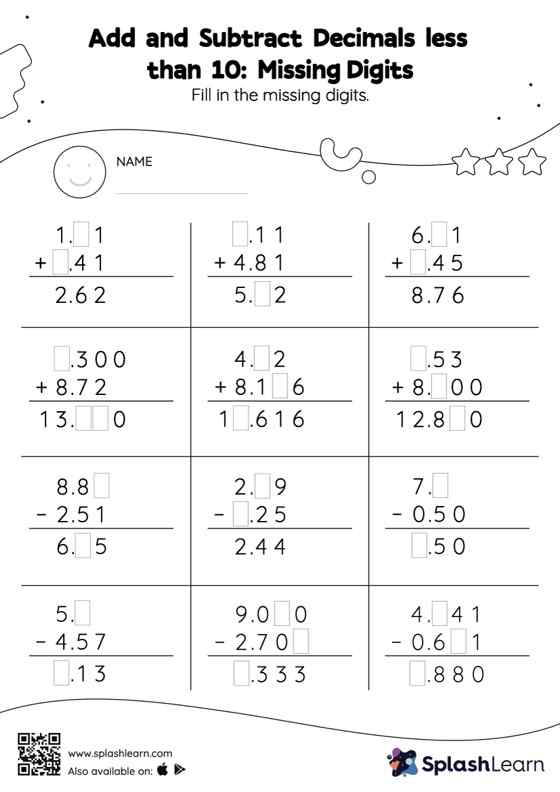# Add and Subtract Decimals less than 10: Missing Digits Worksheet

Home > Add and Subtract Decimals less than 10: Missing DigitsAdd and subtract decimals less than 10 worksheet is a great way to help learners become fluent with the concept of addition and subtraction. This worksheet is about practicing the column method in which numbers are written one on top of another. In this method, students use their place value understanding to solve the problems.